# 240 373 Image Processing Montri Karnjanadecha montricoe psu

• Slides: 19
Download presentation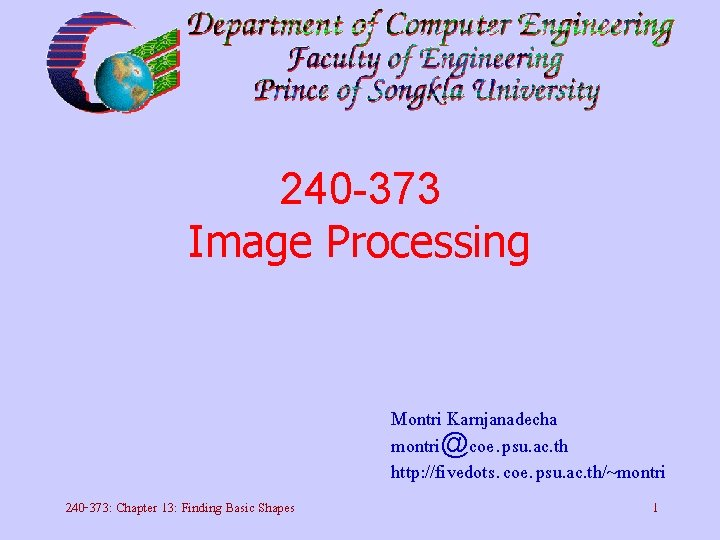240 -373 Image Processing Montri Karnjanadecha [email protected] psu. ac. th http: //fivedots. coe. psu. ac. th/~montri 240 -373: Chapter 13: Finding Basic Shapes 1Chapter 13 Finding Basic Shapes 240 -373: Chapter 13: Finding Basic Shapes 2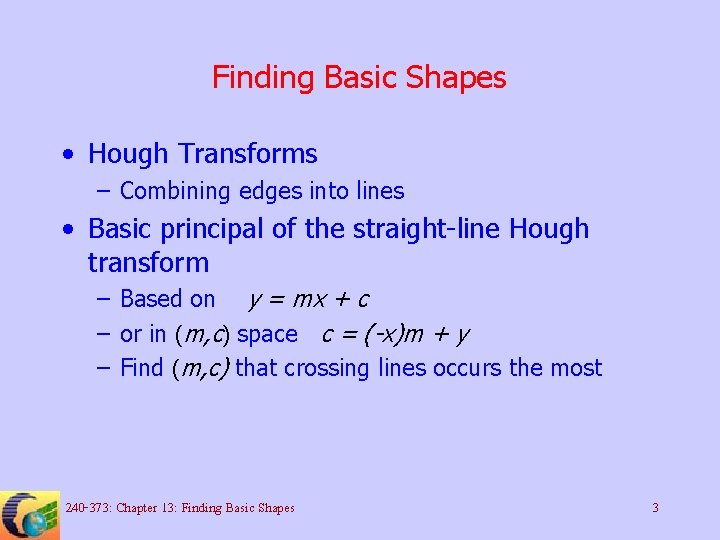Finding Basic Shapes • Hough Transforms – Combining edges into lines • Basic principal of the straight-line Hough transform – Based on y = mx + c – or in (m, c) space c = (-x)m + y – Find (m, c) that crossing lines occurs the most 240 -373: Chapter 13: Finding Basic Shapes 3Finding Basic Shapes 240 -373: Chapter 13: Finding Basic Shapes 4Real straight-edge discovery using the Hough transform • Problem with the basic technique: m could be from -infinity to +infinity • Polar coordinates can be used instead of cartesian coordinates 240 -373: Chapter 13: Finding Basic Shapes 5Real straight-edge discovery using the Hough transform 240 -373: Chapter 13: Finding Basic Shapes 6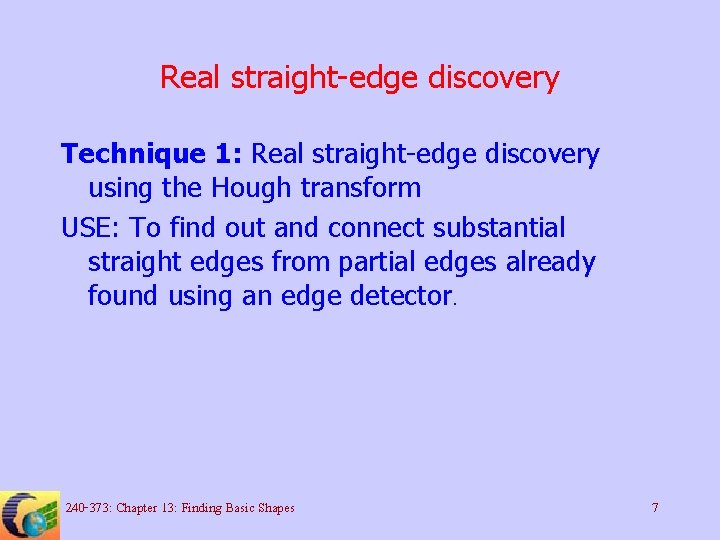Real straight-edge discovery Technique 1: Real straight-edge discovery using the Hough transform USE: To find out and connect substantial straight edges from partial edges already found using an edge detector. 240 -373: Chapter 13: Finding Basic Shapes 7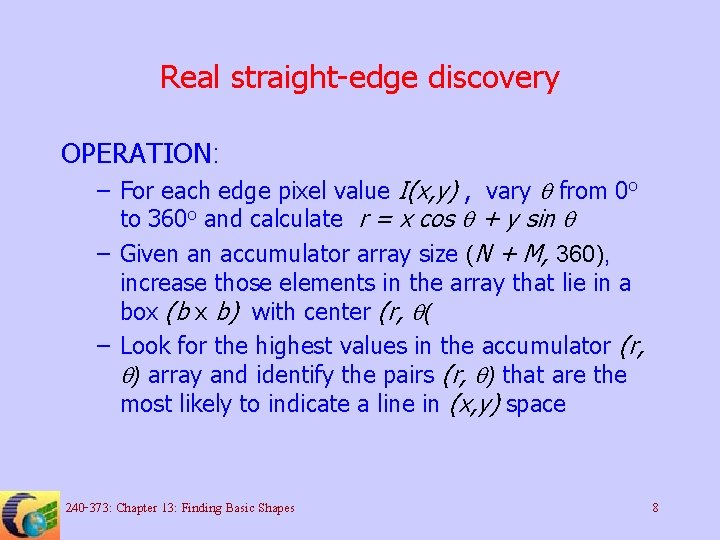Real straight-edge discovery OPERATION: – For each edge pixel value I(x, y) , vary q from 0 o to 360 o and calculate r = x cos q + y sin q – Given an accumulator array size (N + M, 360), increase those elements in the array that lie in a box (b x b) with center (r, q( – Look for the highest values in the accumulator (r, q) array and identify the pairs (r, q) that are the most likely to indicate a line in (x, y) space 240 -373: Chapter 13: Finding Basic Shapes 8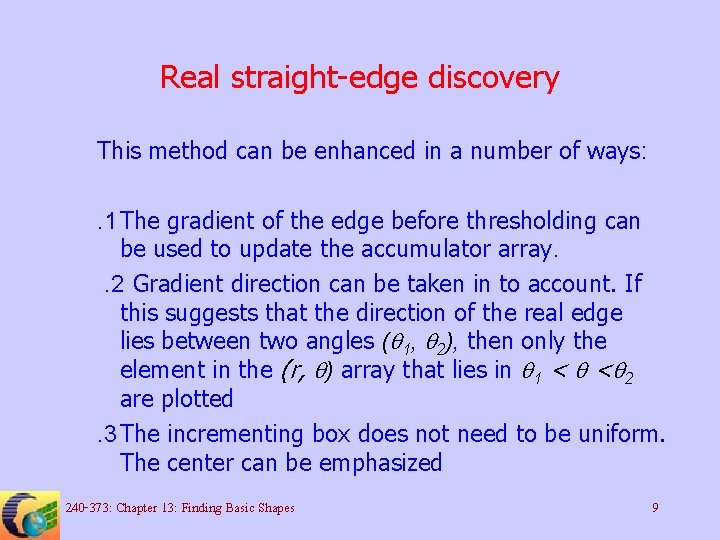Real straight-edge discovery This method can be enhanced in a number of ways: . 1 The gradient of the edge before thresholding can be used to update the accumulator array. . 2 Gradient direction can be taken in to account. If this suggests that the direction of the real edge lies between two angles (q 1, q 2), then only the element in the (r, q) array that lies in q 1 < q <q 2 are plotted. 3 The incrementing box does not need to be uniform. The center can be emphasized 240 -373: Chapter 13: Finding Basic Shapes 9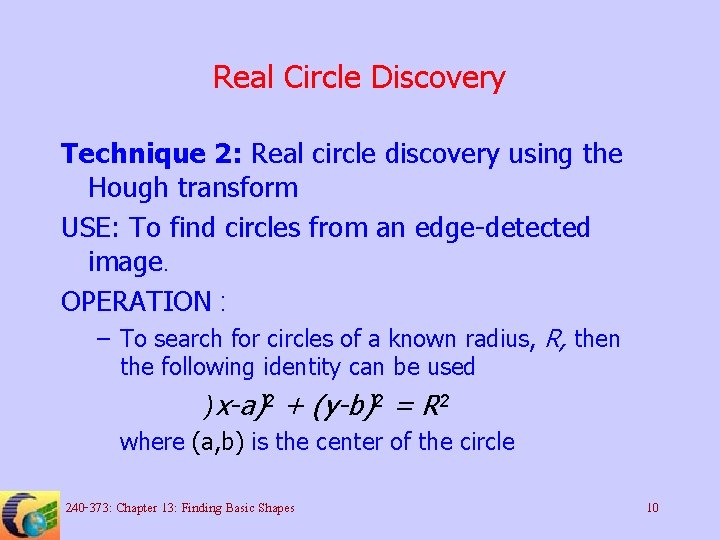Real Circle Discovery Technique 2: Real circle discovery using the Hough transform USE: To find circles from an edge-detected image. OPERATION : – To search for circles of a known radius, R, then the following identity can be used )x-a)2 + (y-b)2 = R 2 where (a, b) is the center of the circle 240 -373: Chapter 13: Finding Basic Shapes 10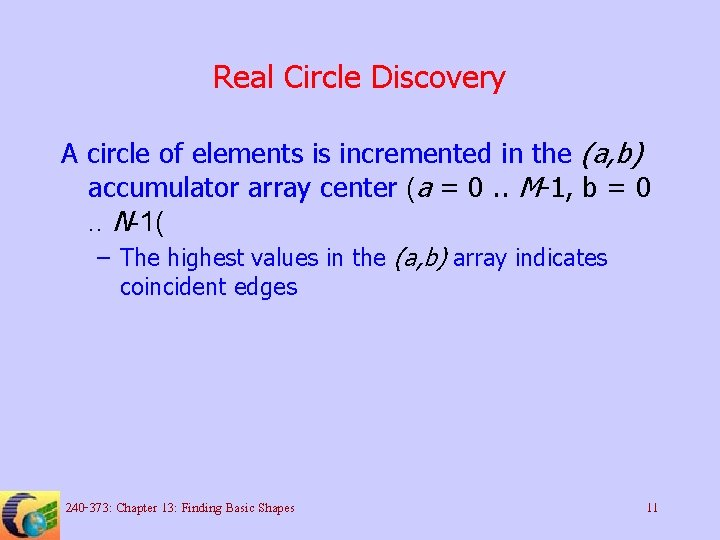Real Circle Discovery A circle of elements is incremented in the (a, b) accumulator array center (a = 0. . M-1, b = 0. . N-1( – The highest values in the (a, b) array indicates coincident edges 240 -373: Chapter 13: Finding Basic Shapes 11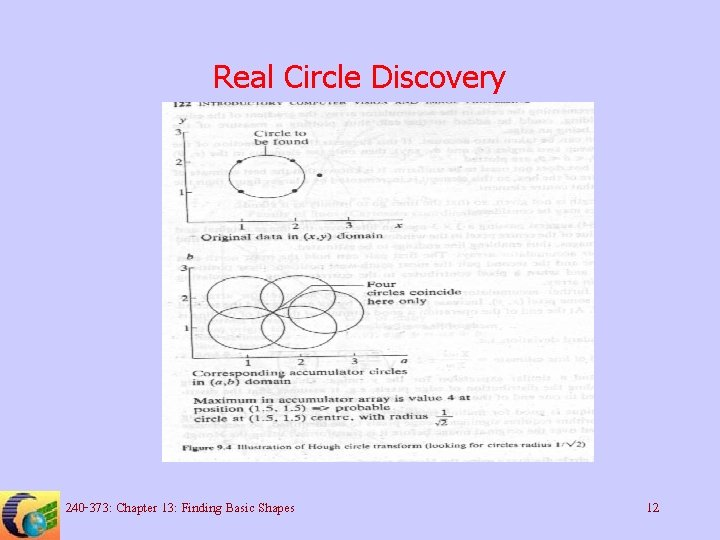Real Circle Discovery 240 -373: Chapter 13: Finding Basic Shapes 12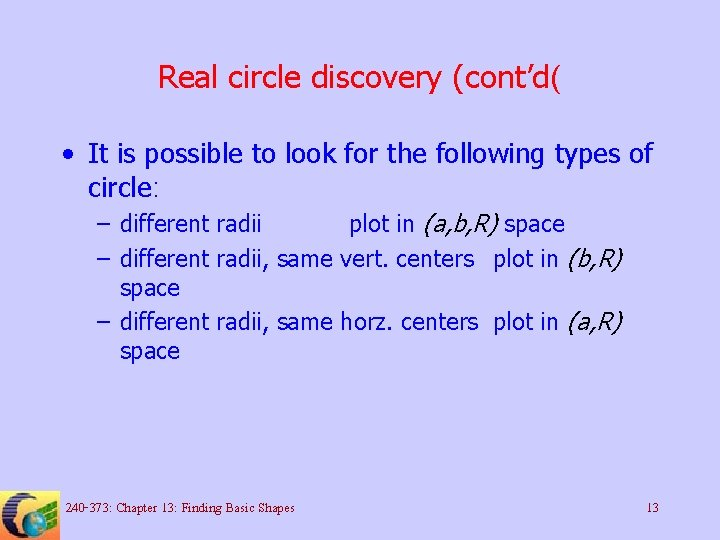Real circle discovery (cont’d( • It is possible to look for the following types of circle: – different radii plot in (a, b, R) space – different radii, same vert. centers plot in (b, R) space – different radii, same horz. centers plot in (a, R) space 240 -373: Chapter 13: Finding Basic Shapes 13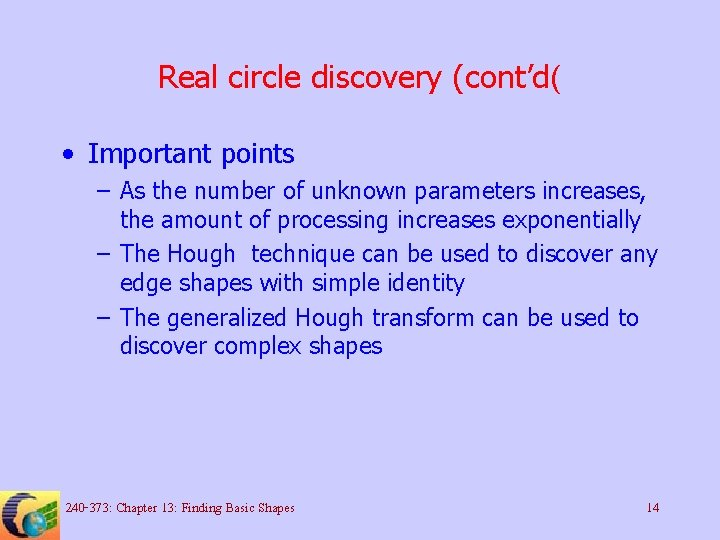Real circle discovery (cont’d( • Important points – As the number of unknown parameters increases, the amount of processing increases exponentially – The Hough technique can be used to discover any edge shapes with simple identity – The generalized Hough transform can be used to discover complex shapes 240 -373: Chapter 13: Finding Basic Shapes 14Generalized Hough transform Technique 2: The generalized Hough transform USE: To find a known shape of any size or orientation in an image. OPERATION : – Given the object boundary (assuming that the object is of the same size and orientation), choose a center (x, y( 240 -373: Chapter 13: Finding Basic Shapes 15Generalized Hough transform OPERATION: (cont’d( – The boundary is traversed and after every step d along the boundary the angle of the boundary tangent with respect to horizontal is noted, and the x difference and y difference of the boundary position from the center point are also noted. – See table 240 -373: Chapter 13: Finding Basic Shapes 16Generalized Hough transform 240 -373: Chapter 13: Finding Basic Shapes 17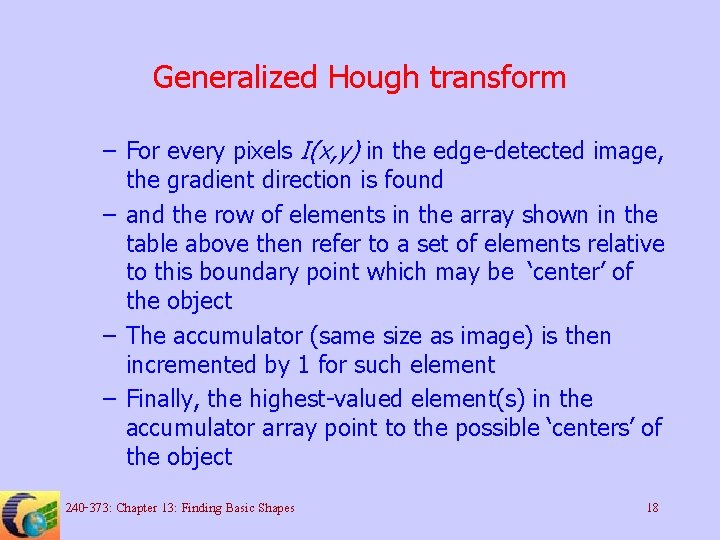Generalized Hough transform – For every pixels I(x, y) in the edge-detected image, the gradient direction is found – and the row of elements in the array shown in the table above then refer to a set of elements relative to this boundary point which may be ‘center’ of the object – The accumulator (same size as image) is then incremented by 1 for such element – Finally, the highest-valued element(s) in the accumulator array point to the possible ‘centers’ of the object 240 -373: Chapter 13: Finding Basic Shapes 18Bresenham’s algorithms • Bresenham’s straight-line drawing algorithm • Bresenham’s circle drawing algorithm 240 -373: Chapter 13: Finding Basic Shapes 19